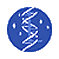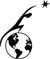Process Theory

In molten silicate electrolysis, metal cations are reduced at the cathode to form metals, and silicate polymer chains are oxidized at the anode to form oxygen. The primary cathode reactions that produce metal are the following: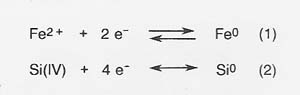The primary anodic reaction producing oxygen is reaction (3).The kinetics of these reactions are fast compared to the current densities expected in actual electrolysis, and reaction kinetics is not a serious constraint on the electrolysis process.

The efficiency of production is decreased and the energy required to produce a given amount of product correspondingly increased because of competing reactions at the anode and cathode. The most serious competing reaction at the anode in melts with high iron concentrations is oxidation of Fe2 + .The efficiency of oxygen production (defined as moles O2 produced/ 4 times moles electrons* passed through the melt) depends primarily on the concentration of Fe2 + cations, with efficiency of oxygen production decreasing as Fe2 + increases.

The electrical conductivity of the melt also has a significant effect on the power requirement of the electrolysis process. We investigated the dependence of melt conductivity on melt composition and found that conductivity increases in a predictable fashion as the proportions of the oxides of silicon and aluminum in the melt decrease and as the proportions of the oxides of iron, magnesium, and calcium increase.

Power Requirements

The power requirement for molten silicate electrolysis can be numerically related to the oxygen production efficiency, the melt conductivity, and the dimensions of the electrolysis cell as follows. Power to drive the electrolysis equals E * I, where I, the current required to get oxygen at the desired rate, is proportional to oxygen production rate/oxygen production efficiency. E, the potential required to drive the electrolysis, is roughly equal to Ec - Ea - I(Rcell), where Ec - Ea, the potential required to drive the reaction(s), is a function of the cation reduced (the absolute value of Ec - Ea increases in the order Fe < Si, Ti < Mg, AI < Ca) and the concentrations of the cations in the melt. Rcell. the resistance of the electrolysis cell, is equal to UKA, where L is the distance between electrodes, A is the electrode surface area, and K is the melt conductivity.

Calculated from this relationship and those above, the energy requirements expected for a realistic range of the variables L, A, O2 eft, and K are shown in figure 22. A comparison is made to energy requirements estimated for other processes. The estimated energy requirements for the molten silicate electrolysis process compare favorably with those for other processes even at the less favorable end of the range for the selected critical variables.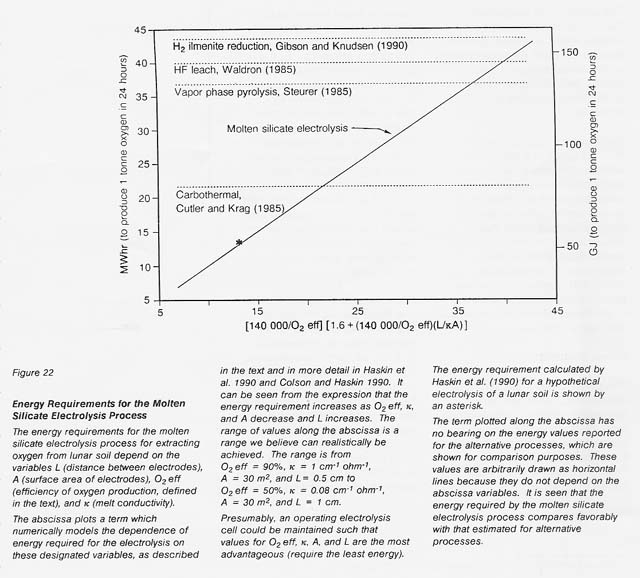*A mole of electrons is Avogodro's number (6.023 x 1023) of electrons.

Next

Table of Contents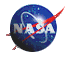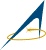WebWork: Al Globus, Bryan Yager, and Tugrul Sezen